# Aim 24 How can we use electrical energy

• Slides: 16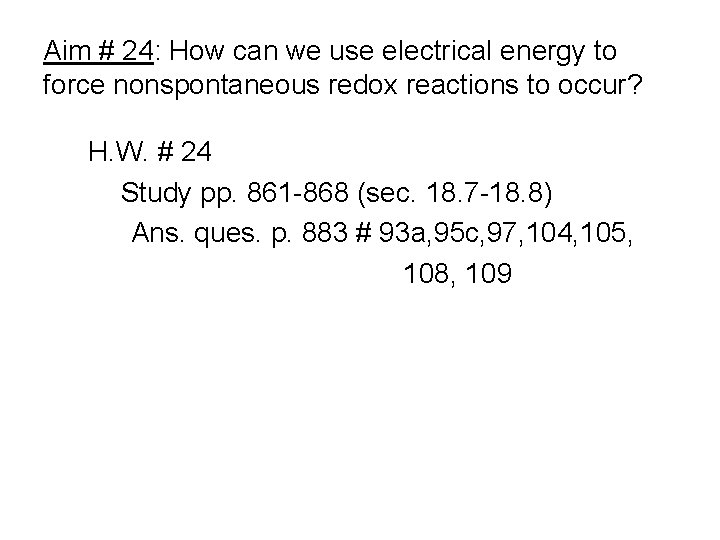Aim # 24: How can we use electrical energy to force nonspontaneous redox reactions to occur? H. W. # 24 Study pp. 861 -868 (sec. 18. 7 -18. 8) Ans. ques. p. 883 # 93 a, 95 c, 97, 104, 105, 108, 109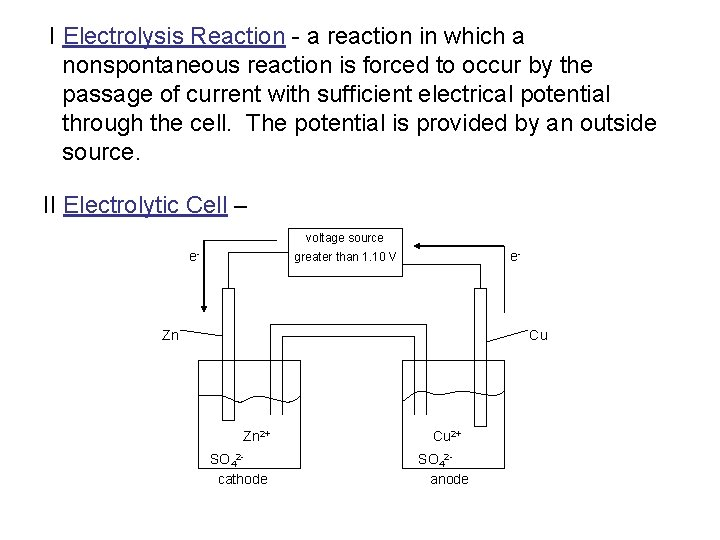I Electrolysis Reaction - a reaction in which a nonspontaneous reaction is forced to occur by the passage of current with sufficient electrical potential through the cell. The potential is provided by an outside source. II Electrolytic Cell – voltage source e- e- greater than 1. 10 V Zn Cu Zn 2+ SO 42 cathode Cu 2+ SO 42 anode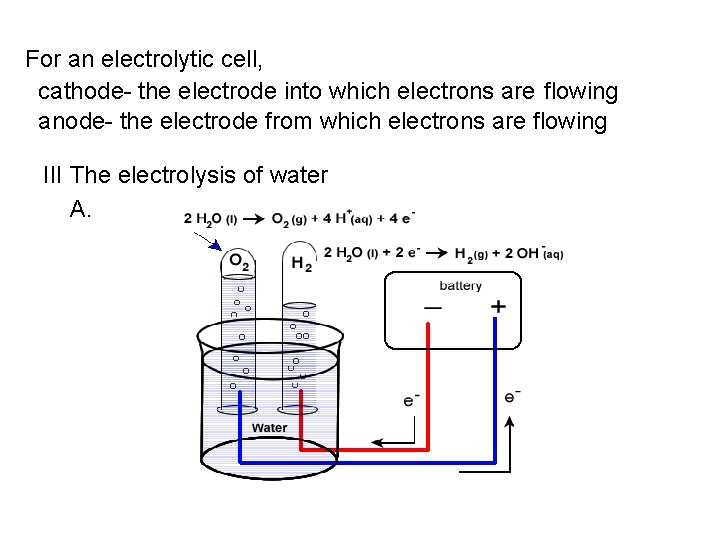For an electrolytic cell, cathode- the electrode into which electrons are flowing anode- the electrode from which electrons are flowing III The electrolysis of water A.Anode reaction: H 2 O → ½ O 2 + 2 H+ + 2 e. Cathode reaction: 2 H 2 O + 2 e- → H 2 + 2 OH- ε 0 = + 1. 23 V ε 0 = - 0. 83 V 3 H 2 O → H 2 + ½ O 2 + (2 H+ + 2 OH-) ε 0 = -2. 06 V OR H 2 O → H 2 + ½ O 2 NOTE: 2 mol of e- are needed to produce 1 mol H 2. How many mol e- are needed to produce 1 mol O 2? Note: a small amount of electrolyte must be added to the water for its electrolysis to occur (so that current will flow), because water contains so few electrons.B. The electrolysis of aqueous solutions 1. In an electrolytic cell, you must consider whether the water or the solute will be oxidized or reduced. Consider, + e- voltage - e- source Pt (anode) ox. Pt (cathode) red. Na. Cl (aq) Cl - Na+ The possible half-reactions are Cathode: Na+(aq) + e- → Na(s) ε 0 = -2. 71 V and 2 H 2 O(ℓ) +2 e- → 2 H 2(g) + 2 OH- ε 0 = -0. 83 V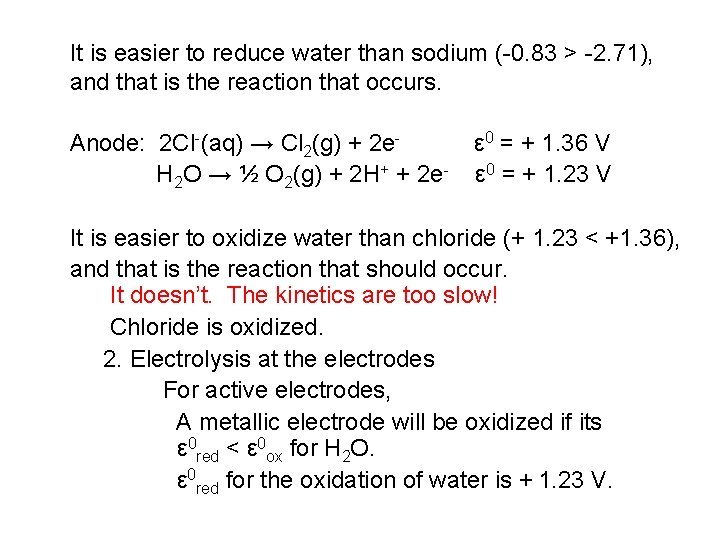It is easier to reduce water than sodium (-0. 83 > -2. 71), and that is the reaction that occurs. Anode: 2 Cl-(aq) → Cl 2(g) + 2 e. H 2 O → ½ O 2(g) + 2 H+ + 2 e- ε 0 = + 1. 36 V ε 0 = + 1. 23 V It is easier to oxidize water than chloride (+ 1. 23 < +1. 36), and that is the reaction that should occur. It doesn’t. The kinetics are too slow! Chloride is oxidized. 2. Electrolysis at the electrodes For active electrodes, A metallic electrode will be oxidized if its ε 0 red < ε 0 ox for H 2 O. ε 0 red for the oxidation of water is + 1. 23 V.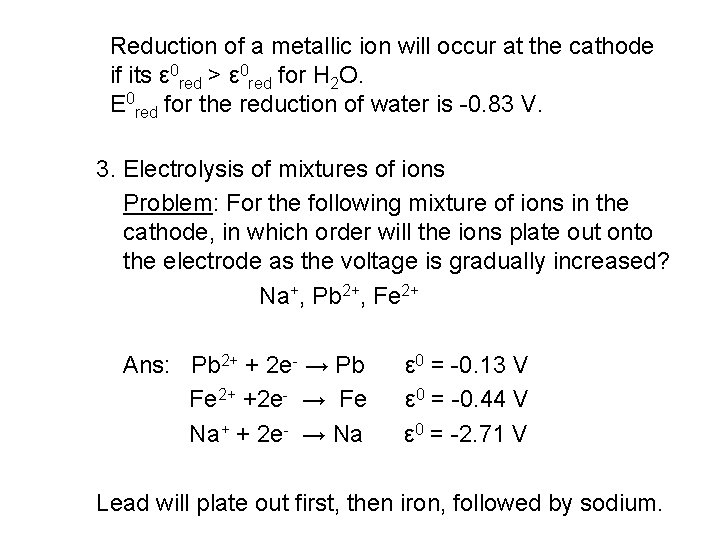Reduction of a metallic ion will occur at the cathode if its ε 0 red > ε 0 red for H 2 O. Ε 0 red for the reduction of water is -0. 83 V. 3. Electrolysis of mixtures of ions Problem: For the following mixture of ions in the cathode, in which order will the ions plate out onto the electrode as the voltage is gradually increased? Na+, Pb 2+, Fe 2+ Ans: Pb 2+ + 2 e- → Pb Fe 2+ +2 e- → Fe Na+ + 2 e- → Na ε 0 = -0. 13 V ε 0 = -0. 44 V ε 0 = -2. 71 V Lead will plate out first, then iron, followed by sodium.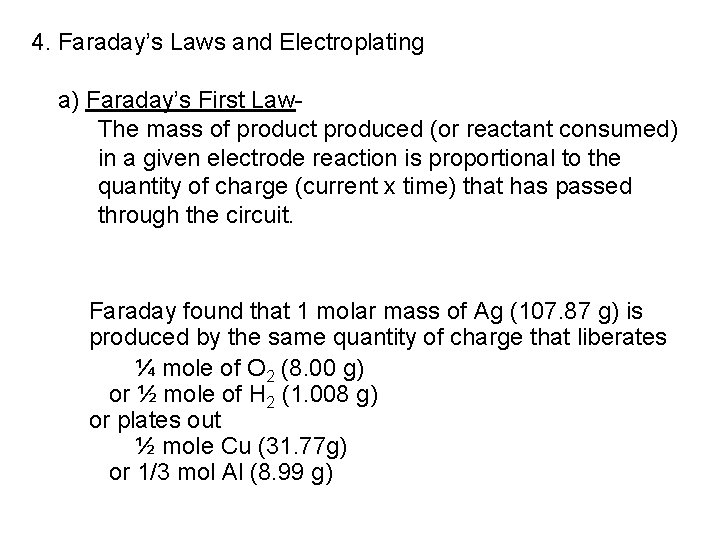4. Faraday’s Laws and Electroplating a) Faraday’s First Law. The mass of product produced (or reactant consumed) in a given electrode reaction is proportional to the quantity of charge (current x time) that has passed through the circuit. Faraday found that 1 molar mass of Ag (107. 87 g) is produced by the same quantity of charge that liberates ¼ mole of O 2 (8. 00 g) or ½ mole of H 2 (1. 008 g) or plates out ½ mole Cu (31. 77 g) or 1/3 mol Al (8. 99 g)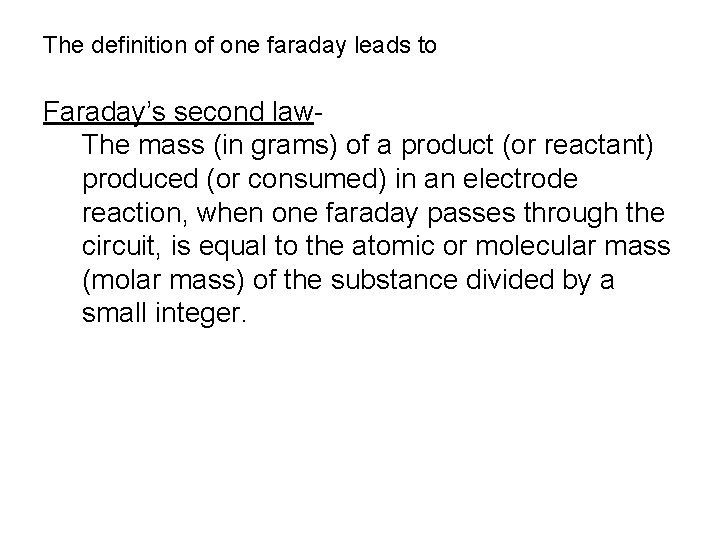The definition of one faraday leads to Faraday’s second law. The mass (in grams) of a product (or reactant) produced (or consumed) in an electrode reaction, when one faraday passes through the circuit, is equal to the atomic or molecular mass (molar mass) of the substance divided by a small integer.Problem: The half-reaction for the formation of magnesium metal upon electrolysis of molten Mg. Cl 2 is Mg 2+ + 2 e- → Mg. Calculate the mass of magnesium formed upon the passage of current at 60. 0 A for a period of 1 hr. Ans: Current: 1 Ampere (A) = 1 coulomb (C)/second (s) Current (A) x time(s) → charge(C) → moles e- → moles Mg → mass Mg (60. 0 C/s)(3600 s) = 216000 C ↑ 1 hr = 3600 s (216000 C)(1 mol e-/96485 C) = 2. 238 mol e-(2. 238 mol e-)(1 mol Mg/2 mol e-) = 1. 12 mol Mg (1. 12 mol Mg)(24. 31 g Mg/ mol Mg) = 27. 2 g Mg Problem: Calculate the time required to produce 3. 44 g I 2 at the anode if a current of 1. 56 A is passed through KI(aq). Ans: mass I 2→moles I 2→mol e- required→C required→time req. (3. 44 g I 2)(1 mol I 2/253. 8 g I 2)(2 mol e-/mol I 2)(96485 C/mol e-)(1 s/1. 56 C) = 1676. 87 s = 27. 9 minProblem: A “deep discharge” lead-acid battery for marine use is advertised as having an 80 -A-hr capacity. What amount of Pb would be oxidized if this battery were discharged so as to consume 80% of its capacity? Ans: The reaction of the Pb electrode is Pb → Pb 2+ + 2 etherefore, 2 F consumes 1 mol Pb. Total available charge from battery = (1 C/s)(3600 s/hr)(80. hr) Coulombs used = (. 80)(1 C/s)(3600 s/hr)(80. hr) = 2. 3 x 105 C Faradays used = (2. 3 x 105 C)(1 F/96500 C) = 2. 38 F Mass of Pb consumed = (2. 38 F)(1 mol Pb/2 F)(207. 2 g Pb/mol Pb) = 250 g PbProblem: An unknown metal from a soluble compound M(NO 3)2 is electrolyzed. When a constant current of 2. 50 A is applied for 35. 0 minutes, 3. 06 g of the metal is deposited. Calculate the molar mass of M and identify the metal. Ans: (2. 50 C/s)(35. 0 min)(60 s/min) = 5. 25 x 103 C (5. 25 x 103 C)(1 mol e-/96500 C) = 0. 0544 mol e. Since M 2+ + 2 e- → M , (0. 0544 mol e-)(1 mol M/2 mol e-) = 0. 0272 mol M 3. 06 g/0. 0272 mol ≈ 112. 5 g /mol Examining the periodic table, M is Cd.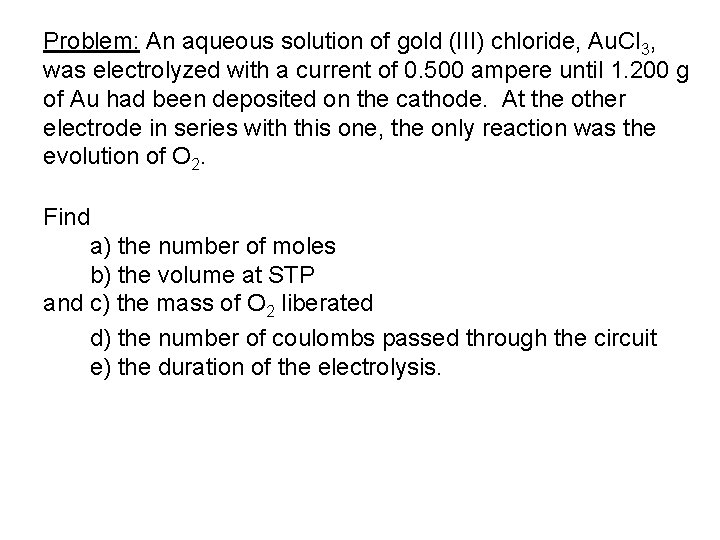Problem: An aqueous solution of gold (III) chloride, Au. Cl 3, was electrolyzed with a current of 0. 500 ampere until 1. 200 g of Au had been deposited on the cathode. At the other electrode in series with this one, the only reaction was the evolution of O 2. Find a) the number of moles b) the volume at STP and c) the mass of O 2 liberated d) the number of coulombs passed through the circuit e) the duration of the electrolysis.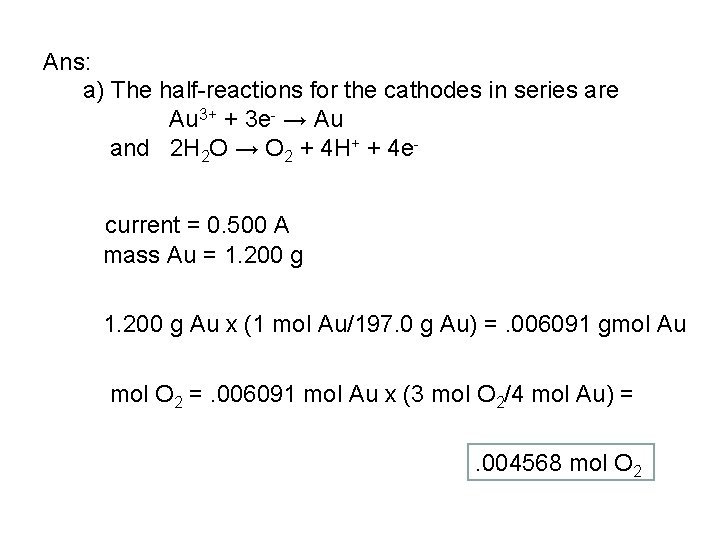Ans: a) The half-reactions for the cathodes in series are Au 3+ + 3 e- → Au and 2 H 2 O → O 2 + 4 H+ + 4 ecurrent = 0. 500 A mass Au = 1. 200 g Au x (1 mol Au/197. 0 g Au) =. 006091 gmol Au mol O 2 =. 006091 mol Au x (3 mol O 2/4 mol Au) =. 004568 mol O 2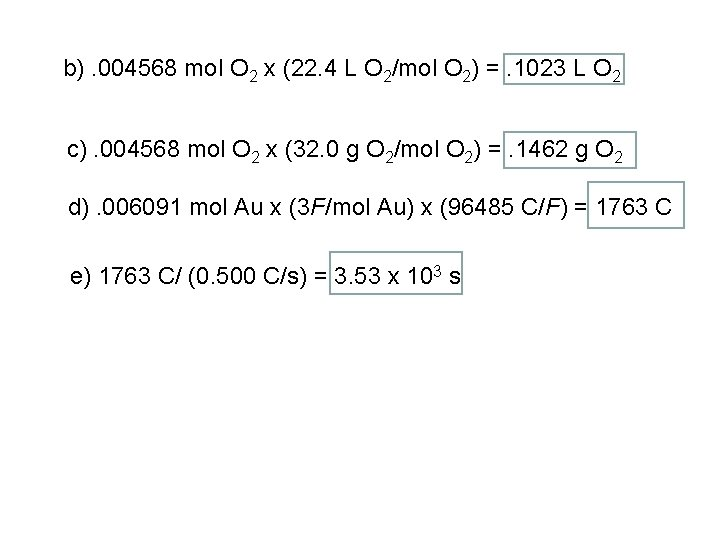b). 004568 mol O 2 x (22. 4 L O 2/mol O 2) =. 1023 L O 2 c). 004568 mol O 2 x (32. 0 g O 2/mol O 2) =. 1462 g O 2 d). 006091 mol Au x (3 F/mol Au) x (96485 C/F) = 1763 C e) 1763 C/ (0. 500 C/s) = 3. 53 x 103 s